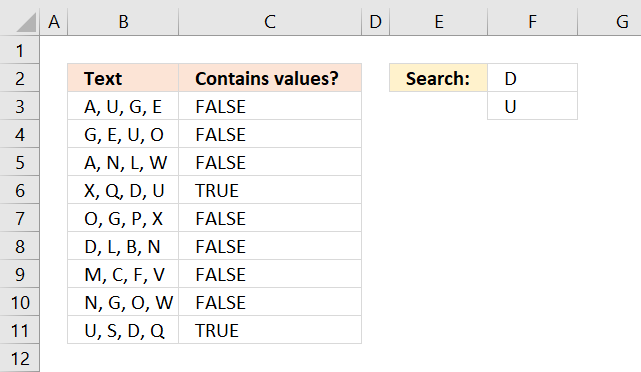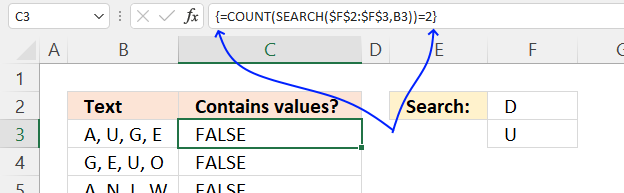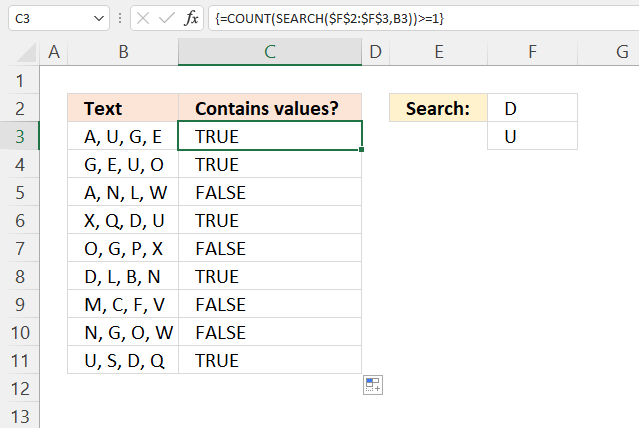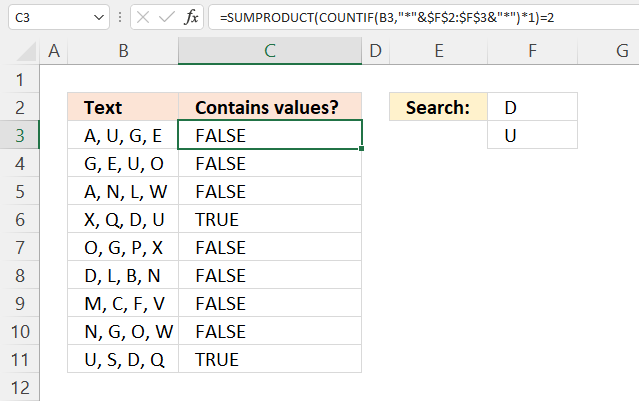Author: Oscar Cronquist Article last updated on January 01, 2022This article demonstrates formulas that perform a partial match for a given cell using multiple strings specified in cells F2 and F3.

## 1. Find cells containing all conditionsThe array formula in cell C3 checks if the value in cell B3 contains all conditions specified in cells F2:F3, it returns a boolean value TRUE or FALSE.

Both conditions must be found in cell C3 in order to return TRUE. For example, cell B4 contains one of the two conditions, however, the formula returns FALSE in cell C4.

=COUNT(SEARCH(\$F\$2:\$F\$3,B3))=2

### 1.1 How to enter an array formulaTo enter an array formula, type the formula in a cell then press and hold CTRL + SHIFT simultaneously, now press Enter once. Release all keys.

The formula bar now shows the formula with a beginning and ending curly bracket telling you that you entered the formula successfully. Don't enter the curly brackets yourself.

### 1.2 Explaining formula in cell C3

#### Step 1 - Find cells containing at least one condition

The SEARCH function returns the character position of a substring in a text string, it returns an error if not found.

SEARCH(find_text,within_text, [start_num])

SEARCH(\$F\$2:\$F\$3,B3)

becomes

SEARCH({"D";"U"},"A, U, G, E")

and returns {#VALUE!;4}.

#### Step 2 - Count numbers in array

The COUNT function counts the number of values that contain a number, it conveniently also ignores errors.

COUNT(value1, [value2], ...)

COUNT(SEARCH(\$F\$2:\$F\$3,B3))

becomes

COUNT({#VALUE!; 4})

and returns 1. Only one value in the array is a number.

#### Step 2 - Count numbers in the array

Lastly, the equal sign compares the output with number 2. There are two conditions specified in cells F2 and F3, this is why the count is compared to two.

COUNT(SEARCH(\$F\$2:\$F\$3,B3))=2

becomes

1=2

and returns FALSE in cell C3.

## 2. Find cells containing at least one conditionIf you want the formula to return TRUE if at least one value is found change the array formula to:

=COUNT(SEARCH(\$F\$2:\$F\$3,B3))=>1

How to enter an array formula

The formula above is almost identical to the formula in section 1, however, there are two comparison operators in this formula instead of one.

The equal sign and the larger than sign combined lets you check if a value is equal to or larger than a given condition. Read section 1.2 for a more detailed explanation.

The possibilities are endless here if you want the formula to return TRUE if at least 2 out of 3 values are found, change the formula to:

=COUNT(SEARCH(\$F\$2:\$F\$4,B3))=>2

## 3. Find cells containing all conditions (regular formula)This formula is slightly larger but has the advantage of being a regular formula, no need to enter the formula as an array formula.

=SUMPRODUCT(COUNTIF(B3,"*"&\$F\$2:\$F\$3&"*")*1)=2

### Explaining formula in cell C3

#### Step 1 - Append asterisks to each condition

The ampersand character lets you concatenate strings in an Excel formula. The asterisk character is a wildcard character that matches 0 (zero) to any length of characters.

The part shown below appends asterisks to the start and end of each string in cells F2 and F3.

"*"&\$F\$2:\$F\$3&"*"

returns

{"*D*"; "*U*"}.

#### Step 2 - Count values using partial match

The COUNTIF function calculates the number of cells that is equal to a condition.

COUNTIF(rangecriteria)

COUNTIF(B3,"*"&\$F\$2:\$F\$3&"*")

becomes

COUNTIF(B3, {"*D*"; "*U*"})

becomes

COUNTIF("A, U, G, E", {"*D*"; "*U*"})

and returns {0; 1}.

#### Step 3 - Multiply by 1

This step is required to convert the array formula to a regular formula, this step is not needed if you are using Excel 365.

COUNTIF(B3,"*"&\$F\$2:\$F\$3&"*")*1

becomes

{0; 1}*1

and returns {0; 1}.

#### Step 4 - Add numbers in array and return a total

The SUMPRODUCT function calculates the product of corresponding values and then returns the sum of each multiplication.

SUMPRODUCT(array1, [array2], ...)

SUMPRODUCT(COUNTIF(B3,"*"&\$F\$2:\$F\$3&"*")*1)

becomes

SUMPRODUCT({0; 1})

and returns 1.

#### Step 5 - Compare the result to two

The equal sign compare the result to two, this makes the formula return TRUE if both string are found. Change this value if you have more or fewer conditions.

SUMPRODUCT(COUNTIF(B3,"*"&\$F\$2:\$F\$3&"*")*1)=2

becomes

1=2

and returns FALSE in cell C3.